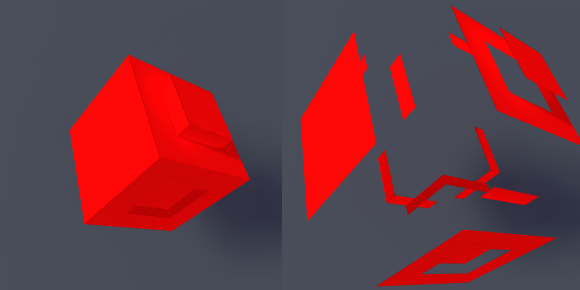Since the topic of GPU’s being optimized for math has been touched upon this next section will demonstrate a method of quickly altering mesh geometry for visual effect. More specifically this shader will cause the faces of a mesh to move along the direction of the surface normals.

The Properties code block for this shader only contains two values, a texture and a ranged float. The ranged float value will be what causes the displacement of the mesh geometry.

Within the SubShader block the #pragma must be extended to contain our new vertex function by appending vertex:vert to the end of this line. The vertex identifier tells the shader compiler that this function will operate on the mesh’s vertexes, and vert simply refers to the new function name. Appending this function the end of #pragma means it will be the last function to be called in this shader, so after the mesh has been prepared to be rendered.

The vert function contains a single inout parameter appdata_full which contains the mesh geometry we want to alter in this case. Within this function each mesh vertex is accessed sequentially then the position of that vertex is added to the scaled vector of the vertex normal by the Amount property. The end result being that the vertex is displaced like we wanted.

```Shader "Custom/ShaderExperiment3" {
Properties {
_MainTex ("Texture", 2D) = "white" {}
_Amount ("Extrusion Amount", Range(-2, 2)) = 0
}
Tags { "RenderType" = "Opaque" }
CGPROGRAM
#pragma surface surf Lambert vertex:vert
struct Input {
float2 uv_MainTex;
};
float _Amount;
void vert(inout appdata_full v)
{
v.vertex.xyz += v.normal * _Amount;
}

sampler2D _MainTex;
void surf (Input IN, inout SurfaceOutput o) {
o.Albedo = tex2D(_MainTex, IN.uv_MainTex).rgb;
}
ENDCG
}
FallBack "Diffuse"
}

```